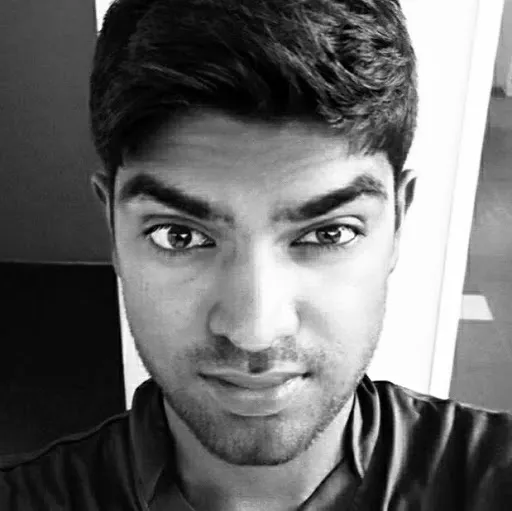# Park&Go: Smart Parking System for Vehicles

This computer vision-based parking system makes vehicle parking process super easy.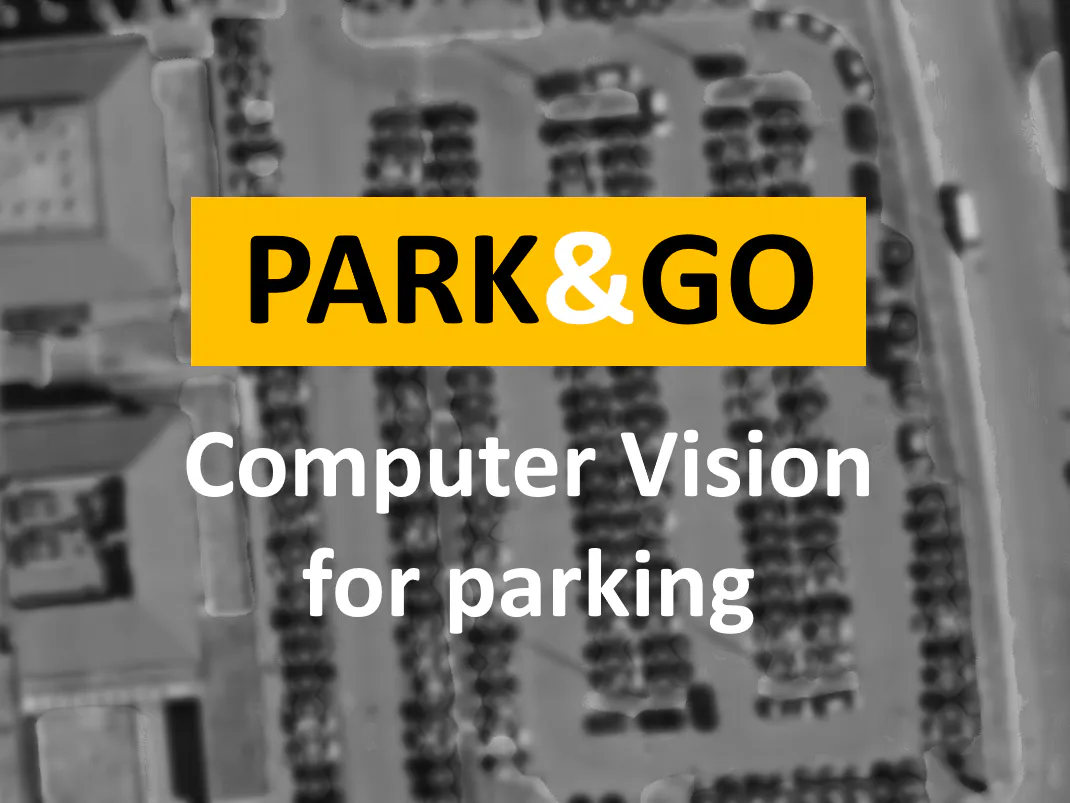## Things used in this project

### Hardware componentsRaspberry Pi 3 Model B
×1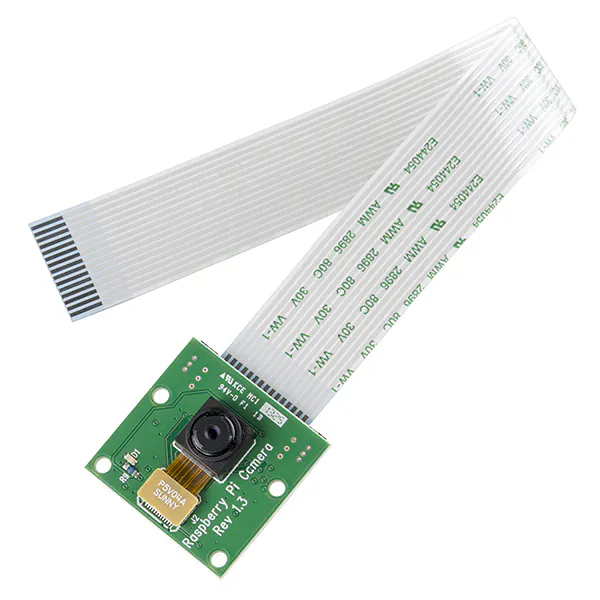Raspberry Pi Camera Module
×1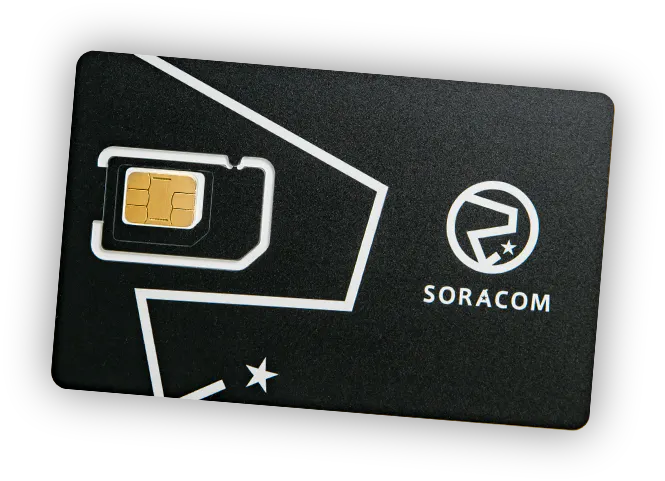SORACOM Air Global IoT SIM Included with SORACOM Basic Starter Kit
×1
 Huawei 3G USB dongle (MS2131i) Included with SORACOM Basic Starter Kit
×1Ultrasonic Sensor - HC-SR04 (Generic)
×1

### Software apps and online services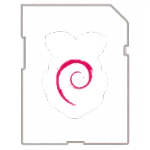Raspberry Pi RaspbianMicrosoft VS Code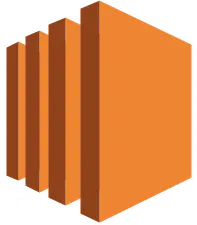Amazon Web Services AWS EC2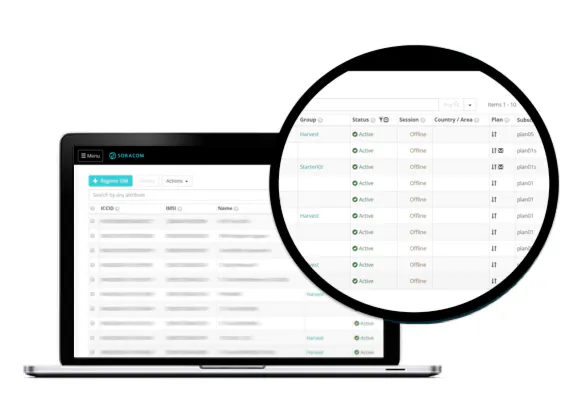SORACOM Air IoT Connectivity Platform
 Ideamart API

### Hand tools and fabrication machines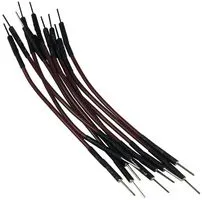10 Pc. Jumper Wire Kit, 5 cm Long

## Code

### DetectPlates.py

Python
```# DetectPlates.py

import cv2
import numpy as np
import math
import Main
import random

import Preprocess
import DetectChars
import PossiblePlate
import PossibleChar

# module level variables ##########################################################################

###################################################################################################
def detectPlatesInScene(imgOriginalScene):
listOfPossiblePlates = []                   # this will be the return value

height, width, numChannels = imgOriginalScene.shape

imgGrayscaleScene = np.zeros((height, width, 1), np.uint8)
imgThreshScene = np.zeros((height, width, 1), np.uint8)
imgContours = np.zeros((height, width, 3), np.uint8)

cv2.destroyAllWindows()

if Main.showSteps == True: # show steps #######################################################
cv2.imshow("0", imgOriginalScene)
# end if # show steps #########################################################################

imgGrayscaleScene, imgThreshScene = Preprocess.preprocess(imgOriginalScene)         # preprocess to get grayscale and threshold images

if Main.showSteps == True: # show steps #######################################################
cv2.imshow("1a", imgGrayscaleScene)
cv2.imshow("1b", imgThreshScene)
# end if # show steps #########################################################################

# find all possible chars in the scene,
# this function first finds all contours, then only includes contours that could be chars (without comparison to other chars yet)
listOfPossibleCharsInScene = findPossibleCharsInScene(imgThreshScene)

if Main.showSteps == True: # show steps #######################################################
print("step 2 - len(listOfPossibleCharsInScene) = " + str(
len(listOfPossibleCharsInScene)))  # 131 with MCLRNF1 image

imgContours = np.zeros((height, width, 3), np.uint8)

contours = []

for possibleChar in listOfPossibleCharsInScene:
contours.append(possibleChar.contour)
# end for

cv2.drawContours(imgContours, contours, -1, Main.SCALAR_WHITE)
cv2.imshow("2b", imgContours)
# end if # show steps #########################################################################

# given a list of all possible chars, find groups of matching chars
# in the next steps each group of matching chars will attempt to be recognized as a plate
listOfListsOfMatchingCharsInScene = DetectChars.findListOfListsOfMatchingChars(listOfPossibleCharsInScene)

if Main.showSteps == True: # show steps #######################################################
print("step 3 - listOfListsOfMatchingCharsInScene.Count = " + str(
len(listOfListsOfMatchingCharsInScene)))  # 13 with MCLRNF1 image

imgContours = np.zeros((height, width, 3), np.uint8)

for listOfMatchingChars in listOfListsOfMatchingCharsInScene:
intRandomBlue = random.randint(0, 255)
intRandomGreen = random.randint(0, 255)
intRandomRed = random.randint(0, 255)

contours = []

for matchingChar in listOfMatchingChars:
contours.append(matchingChar.contour)
# end for

cv2.drawContours(imgContours, contours, -1, (intRandomBlue, intRandomGreen, intRandomRed))
# end for

cv2.imshow("3", imgContours)
# end if # show steps #########################################################################

for listOfMatchingChars in listOfListsOfMatchingCharsInScene:                   # for each group of matching chars
possiblePlate = extractPlate(imgOriginalScene, listOfMatchingChars)         # attempt to extract plate

if possiblePlate.imgPlate is not None:                          # if plate was found
listOfPossiblePlates.append(possiblePlate)                  # add to list of possible plates
# end if
# end for

print("\n" + str(len(listOfPossiblePlates)) + " possible plates found")  # 13 with MCLRNF1 image

if Main.showSteps == True: # show steps #######################################################
print("\n")
cv2.imshow("4a", imgContours)

for i in range(0, len(listOfPossiblePlates)):
p2fRectPoints = cv2.boxPoints(listOfPossiblePlates[i].rrLocationOfPlateInScene)

cv2.line(imgContours, tuple(p2fRectPoints), tuple(p2fRectPoints), Main.SCALAR_RED, 2)
cv2.line(imgContours, tuple(p2fRectPoints), tuple(p2fRectPoints), Main.SCALAR_RED, 2)
cv2.line(imgContours, tuple(p2fRectPoints), tuple(p2fRectPoints), Main.SCALAR_RED, 2)
cv2.line(imgContours, tuple(p2fRectPoints), tuple(p2fRectPoints), Main.SCALAR_RED, 2)

cv2.imshow("4a", imgContours)

print("possible plate " + str(i) + ", click on any image and press a key to continue . . .")

cv2.imshow("4b", listOfPossiblePlates[i].imgPlate)
cv2.waitKey(0)
# end for

print("\nplate detection complete, click on any image and press a key to begin char recognition . . .\n")
cv2.waitKey(0)
# end if # show steps #########################################################################

return listOfPossiblePlates
# end function

###################################################################################################
def findPossibleCharsInScene(imgThresh):
listOfPossibleChars = []                # this will be the return value

intCountOfPossibleChars = 0

imgThreshCopy = imgThresh.copy()

imgContours, contours, npaHierarchy = cv2.findContours(imgThreshCopy, cv2.RETR_LIST, cv2.CHAIN_APPROX_SIMPLE)   # find all contours

height, width = imgThresh.shape
imgContours = np.zeros((height, width, 3), np.uint8)

for i in range(0, len(contours)):                       # for each contour

if Main.showSteps == True: # show steps ###################################################
cv2.drawContours(imgContours, contours, i, Main.SCALAR_WHITE)
# end if # show steps #####################################################################

possibleChar = PossibleChar.PossibleChar(contours[i])

if DetectChars.checkIfPossibleChar(possibleChar):                   # if contour is a possible char, note this does not compare to other chars (yet) . . .
intCountOfPossibleChars = intCountOfPossibleChars + 1           # increment count of possible chars
listOfPossibleChars.append(possibleChar)                        # and add to list of possible chars
# end if
# end for

if Main.showSteps == True: # show steps #######################################################
print("\nstep 2 - len(contours) = " + str(len(contours)))  # 2362 with MCLRNF1 image
print("step 2 - intCountOfPossibleChars = " + str(intCountOfPossibleChars))  # 131 with MCLRNF1 image
cv2.imshow("2a", imgContours)
# end if # show steps #########################################################################

return listOfPossibleChars
# end function

###################################################################################################
def extractPlate(imgOriginal, listOfMatchingChars):
possiblePlate = PossiblePlate.PossiblePlate()           # this will be the return value

listOfMatchingChars.sort(key = lambda matchingChar: matchingChar.intCenterX)        # sort chars from left to right based on x position

# calculate the center point of the plate
fltPlateCenterX = (listOfMatchingChars.intCenterX + listOfMatchingChars[len(listOfMatchingChars) - 1].intCenterX) / 2.0
fltPlateCenterY = (listOfMatchingChars.intCenterY + listOfMatchingChars[len(listOfMatchingChars) - 1].intCenterY) / 2.0

ptPlateCenter = fltPlateCenterX, fltPlateCenterY

# calculate plate width and height
intPlateWidth = int((listOfMatchingChars[len(listOfMatchingChars) - 1].intBoundingRectX + listOfMatchingChars[len(listOfMatchingChars) - 1].intBoundingRectWidth - listOfMatchingChars.intBoundingRectX) * PLATE_WIDTH_PADDING_FACTOR)

intTotalOfCharHeights = 0

for matchingChar in listOfMatchingChars:
intTotalOfCharHeights = intTotalOfCharHeights + matchingChar.intBoundingRectHeight
# end for

fltAverageCharHeight = intTotalOfCharHeights / len(listOfMatchingChars)

# calculate correction angle of plate region
fltOpposite = listOfMatchingChars[len(listOfMatchingChars) - 1].intCenterY - listOfMatchingChars.intCenterY
fltHypotenuse = DetectChars.distanceBetweenChars(listOfMatchingChars, listOfMatchingChars[len(listOfMatchingChars) - 1])
fltCorrectionAngleInDeg = fltCorrectionAngleInRad * (180.0 / math.pi)

# pack plate region center point, width and height, and correction angle into rotated rect member variable of plate
possiblePlate.rrLocationOfPlateInScene = ( tuple(ptPlateCenter), (intPlateWidth, intPlateHeight), fltCorrectionAngleInDeg )

# final steps are to perform the actual rotation

# get the rotation matrix for our calculated correction angle
rotationMatrix = cv2.getRotationMatrix2D(tuple(ptPlateCenter), fltCorrectionAngleInDeg, 1.0)

height, width, numChannels = imgOriginal.shape      # unpack original image width and height

imgRotated = cv2.warpAffine(imgOriginal, rotationMatrix, (width, height))       # rotate the entire image

imgCropped = cv2.getRectSubPix(imgRotated, (intPlateWidth, intPlateHeight), tuple(ptPlateCenter))

possiblePlate.imgPlate = imgCropped         # copy the cropped plate image into the applicable member variable of the possible plate

return possiblePlate
# end function
```

### DetectChars.py

Python
```# DetectChars.py
import os

import cv2
import numpy as np
import math
import random

import Main
import Preprocess
import PossibleChar

# module level variables ##########################################################################

kNearest = cv2.ml.KNearest_create()

# constants for checkIfPossibleChar, this checks one possible char only (does not compare to another char)
MIN_PIXEL_WIDTH = 2
MIN_PIXEL_HEIGHT = 8

MIN_ASPECT_RATIO = 0.25
MAX_ASPECT_RATIO = 1.0

MIN_PIXEL_AREA = 80

# constants for comparing two chars
MIN_DIAG_SIZE_MULTIPLE_AWAY = 0.3
MAX_DIAG_SIZE_MULTIPLE_AWAY = 5.0

MAX_CHANGE_IN_AREA = 0.5

MAX_CHANGE_IN_WIDTH = 0.8
MAX_CHANGE_IN_HEIGHT = 0.2

MAX_ANGLE_BETWEEN_CHARS = 12.0

# other constants
MIN_NUMBER_OF_MATCHING_CHARS = 3

RESIZED_CHAR_IMAGE_WIDTH = 20
RESIZED_CHAR_IMAGE_HEIGHT = 30

MIN_CONTOUR_AREA = 100

###################################################################################################
allContoursWithData = []                # declare empty lists,
validContoursWithData = []              # we will fill these shortly

try:
except:                                                                                 # if file could not be opened
print("error, unable to open classifications.txt, exiting program\n")  # show error message
os.system("pause")
return False                                                                        # and return False
# end try

try:
except:                                                                                 # if file could not be opened
print("error, unable to open flattened_images.txt, exiting program\n")  # show error message
os.system("pause")
return False                                                                        # and return False
# end try

npaClassifications = npaClassifications.reshape((npaClassifications.size, 1))       # reshape numpy array to 1d, necessary to pass to call to train

kNearest.setDefaultK(1)                                                             # set default K to 1

kNearest.train(npaFlattenedImages, cv2.ml.ROW_SAMPLE, npaClassifications)           # train KNN object

return True                             # if we got here training was successful so return true
# end function

###################################################################################################
def detectCharsInPlates(listOfPossiblePlates):
intPlateCounter = 0
imgContours = None
contours = []

if len(listOfPossiblePlates) == 0:          # if list of possible plates is empty
return listOfPossiblePlates             # return
# end if

# at this point we can be sure the list of possible plates has at least one plate

for possiblePlate in listOfPossiblePlates:          # for each possible plate, this is a big for loop that takes up most of the function

possiblePlate.imgGrayscale, possiblePlate.imgThresh = Preprocess.preprocess(possiblePlate.imgPlate)     # preprocess to get grayscale and threshold images

if Main.showSteps == True: # show steps ###################################################
cv2.imshow("5a", possiblePlate.imgPlate)
cv2.imshow("5b", possiblePlate.imgGrayscale)
cv2.imshow("5c", possiblePlate.imgThresh)
# end if # show steps #####################################################################

# increase size of plate image for easier viewing and char detection
possiblePlate.imgThresh = cv2.resize(possiblePlate.imgThresh, (0, 0), fx = 1.6, fy = 1.6)

# threshold again to eliminate any gray areas
thresholdValue, possiblePlate.imgThresh = cv2.threshold(possiblePlate.imgThresh, 0.0, 255.0, cv2.THRESH_BINARY | cv2.THRESH_OTSU)

if Main.showSteps == True: # show steps ###################################################
cv2.imshow("5d", possiblePlate.imgThresh)
# end if # show steps #####################################################################

# find all possible chars in the plate,
# this function first finds all contours, then only includes contours that could be chars (without comparison to other chars yet)
listOfPossibleCharsInPlate = findPossibleCharsInPlate(possiblePlate.imgGrayscale, possiblePlate.imgThresh)

if Main.showSteps == True: # show steps ###################################################
height, width, numChannels = possiblePlate.imgPlate.shape
imgContours = np.zeros((height, width, 3), np.uint8)
del contours[:]                                         # clear the contours list

for possibleChar in listOfPossibleCharsInPlate:
contours.append(possibleChar.contour)
# end for

cv2.drawContours(imgContours, contours, -1, Main.SCALAR_WHITE)

cv2.imshow("6", imgContours)
# end if # show steps #####################################################################

# given a list of all possible chars, find groups of matching chars within the plate
listOfListsOfMatchingCharsInPlate = findListOfListsOfMatchingChars(listOfPossibleCharsInPlate)

if Main.showSteps == True: # show steps ###################################################
imgContours = np.zeros((height, width, 3), np.uint8)
del contours[:]

for listOfMatchingChars in listOfListsOfMatchingCharsInPlate:
intRandomBlue = random.randint(0, 255)
intRandomGreen = random.randint(0, 255)
intRandomRed = random.randint(0, 255)

for matchingChar in listOfMatchingChars:
contours.append(matchingChar.contour)
# end for
cv2.drawContours(imgContours, contours, -1, (intRandomBlue, intRandomGreen, intRandomRed))
# end for
cv2.imshow("7", imgContours)
# end if # show steps #####################################################################

if (len(listOfListsOfMatchingCharsInPlate) == 0):			# if no groups of matching chars were found in the plate

if Main.showSteps == True: # show steps ###############################################
print("chars found in plate number " + str(
intPlateCounter) + " = (none), click on any image and press a key to continue . . .")
intPlateCounter = intPlateCounter + 1
cv2.destroyWindow("8")
cv2.destroyWindow("9")
cv2.destroyWindow("10")
cv2.waitKey(0)
# end if # show steps #################################################################

possiblePlate.strChars = ""
# end if

for i in range(0, len(listOfListsOfMatchingCharsInPlate)):                              # within each list of matching chars
listOfListsOfMatchingCharsInPlate[i].sort(key = lambda matchingChar: matchingChar.intCenterX)        # sort chars from left to right
listOfListsOfMatchingCharsInPlate[i] = removeInnerOverlappingChars(listOfListsOfMatchingCharsInPlate[i])              # and remove inner overlapping chars
# end for

if Main.showSteps == True: # show steps ###################################################
imgContours = np.zeros((height, width, 3), np.uint8)

for listOfMatchingChars in listOfListsOfMatchingCharsInPlate:
intRandomBlue = random.randint(0, 255)
intRandomGreen = random.randint(0, 255)
intRandomRed = random.randint(0, 255)

del contours[:]

for matchingChar in listOfMatchingChars:
contours.append(matchingChar.contour)
# end for

cv2.drawContours(imgContours, contours, -1, (intRandomBlue, intRandomGreen, intRandomRed))
# end for
cv2.imshow("8", imgContours)
# end if # show steps #####################################################################

# within each possible plate, suppose the longest list of potential matching chars is the actual list of chars
intLenOfLongestListOfChars = 0
intIndexOfLongestListOfChars = 0

# loop through all the vectors of matching chars, get the index of the one with the most chars
for i in range(0, len(listOfListsOfMatchingCharsInPlate)):
if len(listOfListsOfMatchingCharsInPlate[i]) > intLenOfLongestListOfChars:
intLenOfLongestListOfChars = len(listOfListsOfMatchingCharsInPlate[i])
intIndexOfLongestListOfChars = i
# end if
# end for

# suppose that the longest list of matching chars within the plate is the actual list of chars
longestListOfMatchingCharsInPlate = listOfListsOfMatchingCharsInPlate[intIndexOfLongestListOfChars]

if Main.showSteps == True: # show steps ###################################################
imgContours = np.zeros((height, width, 3), np.uint8)
del contours[:]

for matchingChar in longestListOfMatchingCharsInPlate:
contours.append(matchingChar.contour)
# end for

cv2.drawContours(imgContours, contours, -1, Main.SCALAR_WHITE)

cv2.imshow("9", imgContours)
# end if # show steps #####################################################################

possiblePlate.strChars = recognizeCharsInPlate(possiblePlate.imgThresh, longestListOfMatchingCharsInPlate)

if Main.showSteps == True: # show steps ###################################################
print("chars found in plate number " + str(
intPlateCounter) + " = " + possiblePlate.strChars + ", click on any image and press a key to continue . . .")
intPlateCounter = intPlateCounter + 1
cv2.waitKey(0)
# end if # show steps #####################################################################

# end of big for loop that takes up most of the function

if Main.showSteps == True:
print("\nchar detection complete, click on any image and press a key to continue . . .\n")
cv2.waitKey(0)
# end if

return listOfPossiblePlates
# end function

###################################################################################################
def findPossibleCharsInPlate(imgGrayscale, imgThresh):
listOfPossibleChars = []                        # this will be the return value
contours = []
imgThreshCopy = imgThresh.copy()

# find all contours in plate
imgContours, contours, npaHierarchy = cv2.findContours(imgThreshCopy, cv2.RETR_LIST, cv2.CHAIN_APPROX_SIMPLE)

for contour in contours:                        # for each contour
possibleChar = PossibleChar.PossibleChar(contour)

if checkIfPossibleChar(possibleChar):              # if contour is a possible char, note this does not compare to other chars (yet) . . .
listOfPossibleChars.append(possibleChar)       # add to list of possible chars
# end if
# end if

return listOfPossibleChars
# end function

###################################################################################################
def checkIfPossibleChar(possibleChar):
# this function is a 'first pass' that does a rough check on a contour to see if it could be a char,
# note that we are not (yet) comparing the char to other chars to look for a group
if (possibleChar.intBoundingRectArea > MIN_PIXEL_AREA and
possibleChar.intBoundingRectWidth > MIN_PIXEL_WIDTH and possibleChar.intBoundingRectHeight > MIN_PIXEL_HEIGHT and
MIN_ASPECT_RATIO < possibleChar.fltAspectRatio and possibleChar.fltAspectRatio < MAX_ASPECT_RATIO):
return True
else:
return False
# end if
# end function

###################################################################################################
def findListOfListsOfMatchingChars(listOfPossibleChars):
# with this function, we start off with all the possible chars in one big list
# the purpose of this function is to re-arrange the one big list of chars into a list of lists of matching chars,
# note that chars that are not found to be in a group of matches do not need to be considered further
listOfListsOfMatchingChars = []                  # this will be the return value

for possibleChar in listOfPossibleChars:                        # for each possible char in the one big list of chars
listOfMatchingChars = findListOfMatchingChars(possibleChar, listOfPossibleChars)        # find all chars in the big list that match the current char

listOfMatchingChars.append(possibleChar)                # also add the current char to current possible list of matching chars

if len(listOfMatchingChars) < MIN_NUMBER_OF_MATCHING_CHARS:     # if current possible list of matching chars is not long enough to constitute a possible plate
continue                            # jump back to the top of the for loop and try again with next char, note that it's not necessary
# to save the list in any way since it did not have enough chars to be a possible plate
# end if

# if we get here, the current list passed test as a "group" or "cluster" of matching chars
listOfListsOfMatchingChars.append(listOfMatchingChars)      # so add to our list of lists of matching chars

listOfPossibleCharsWithCurrentMatchesRemoved = []

# remove the current list of matching chars from the big list so we don't use those same chars twice,
# make sure to make a new big list for this since we don't want to change the original big list
listOfPossibleCharsWithCurrentMatchesRemoved = list(set(listOfPossibleChars) - set(listOfMatchingChars))

recursiveListOfListsOfMatchingChars = findListOfListsOfMatchingChars(listOfPossibleCharsWithCurrentMatchesRemoved)      # recursive call

for recursiveListOfMatchingChars in recursiveListOfListsOfMatchingChars:        # for each list of matching chars found by recursive call
listOfListsOfMatchingChars.append(recursiveListOfMatchingChars)             # add to our original list of lists of matching chars
# end for

break       # exit for

# end for

return listOfListsOfMatchingChars
# end function

###################################################################################################
def findListOfMatchingChars(possibleChar, listOfChars):
# the purpose of this function is, given a possible char and a big list of possible chars,
# find all chars in the big list that are a match for the single possible char, and return those matching chars as a list
listOfMatchingChars = []                # this will be the return value

for possibleMatchingChar in listOfChars:                # for each char in big list
if possibleMatchingChar == possibleChar:    # if the char we attempting to find matches for is the exact same char as the char in the big list we are currently checking
# then we should not include it in the list of matches b/c that would end up double including the current char
# end if
# compute stuff to see if chars are a match
fltDistanceBetweenChars = distanceBetweenChars(possibleChar, possibleMatchingChar)

fltAngleBetweenChars = angleBetweenChars(possibleChar, possibleMatchingChar)

fltChangeInArea = float(abs(possibleMatchingChar.intBoundingRectArea - possibleChar.intBoundingRectArea)) / float(possibleChar.intBoundingRectArea)

fltChangeInWidth = float(abs(possibleMatchingChar.intBoundingRectWidth - possibleChar.intBoundingRectWidth)) / float(possibleChar.intBoundingRectWidth)
fltChangeInHeight = float(abs(possibleMatchingChar.intBoundingRectHeight - possibleChar.intBoundingRectHeight)) / float(possibleChar.intBoundingRectHeight)

# check if chars match
if (fltDistanceBetweenChars < (possibleChar.fltDiagonalSize * MAX_DIAG_SIZE_MULTIPLE_AWAY) and
fltAngleBetweenChars < MAX_ANGLE_BETWEEN_CHARS and
fltChangeInArea < MAX_CHANGE_IN_AREA and
fltChangeInWidth < MAX_CHANGE_IN_WIDTH and
fltChangeInHeight < MAX_CHANGE_IN_HEIGHT):

listOfMatchingChars.append(possibleMatchingChar)        # if the chars are a match, add the current char to list of matching chars
# end if
# end for

return listOfMatchingChars                  # return result
# end function

###################################################################################################
# use Pythagorean theorem to calculate distance between two chars
def distanceBetweenChars(firstChar, secondChar):
intX = abs(firstChar.intCenterX - secondChar.intCenterX)
intY = abs(firstChar.intCenterY - secondChar.intCenterY)

return math.sqrt((intX ** 2) + (intY ** 2))
# end function

###################################################################################################
# use basic trigonometry (SOH CAH TOA) to calculate angle between chars
def angleBetweenChars(firstChar, secondChar):
fltOpp = float(abs(firstChar.intCenterY - secondChar.intCenterY))

if fltAdj != 0.0:                           # check to make sure we do not divide by zero if the center X positions are equal, float division by zero will cause a crash in Python
else:
fltAngleInRad = 1.5708                          # if adjacent is zero, use this as the angle, this is to be consistent with the C++ version of this program
# end if

fltAngleInDeg = fltAngleInRad * (180.0 / math.pi)       # calculate angle in degrees

return fltAngleInDeg
# end function

###################################################################################################
# if we have two chars overlapping or to close to each other to possibly be separate chars, remove the inner (smaller) char,
# this is to prevent including the same char twice if two contours are found for the same char,
# for example for the letter 'O' both the inner ring and the outer ring may be found as contours, but we should only include the char once
def removeInnerOverlappingChars(listOfMatchingChars):
listOfMatchingCharsWithInnerCharRemoved = list(listOfMatchingChars)                # this will be the return value

for currentChar in listOfMatchingChars:
for otherChar in listOfMatchingChars:
if currentChar != otherChar:        # if current char and other char are not the same char . . .
# if current char and other char have center points at almost the same location . . .
if distanceBetweenChars(currentChar, otherChar) < (currentChar.fltDiagonalSize * MIN_DIAG_SIZE_MULTIPLE_AWAY):
# if we get in here we have found overlapping chars
# next we identify which char is smaller, then if that char was not already removed on a previous pass, remove it
if currentChar.intBoundingRectArea < otherChar.intBoundingRectArea:         # if current char is smaller than other char
if currentChar in listOfMatchingCharsWithInnerCharRemoved:              # if current char was not already removed on a previous pass . . .
listOfMatchingCharsWithInnerCharRemoved.remove(currentChar)         # then remove current char
# end if
else:                                                                       # else if other char is smaller than current char
if otherChar in listOfMatchingCharsWithInnerCharRemoved:                # if other char was not already removed on a previous pass . . .
listOfMatchingCharsWithInnerCharRemoved.remove(otherChar)           # then remove other char
# end if
# end if
# end if
# end if
# end for
# end for

return listOfMatchingCharsWithInnerCharRemoved
# end function

###################################################################################################
# this is where we apply the actual char recognition
def recognizeCharsInPlate(imgThresh, listOfMatchingChars):
strChars = ""               # this will be the return value, the chars in the lic plate

height, width = imgThresh.shape

imgThreshColor = np.zeros((height, width, 3), np.uint8)

listOfMatchingChars.sort(key = lambda matchingChar: matchingChar.intCenterX)        # sort chars from left to right

cv2.cvtColor(imgThresh, cv2.COLOR_GRAY2BGR, imgThreshColor)                     # make color version of threshold image so we can draw contours in color on it

for currentChar in listOfMatchingChars:                                         # for each char in plate
pt1 = (currentChar.intBoundingRectX, currentChar.intBoundingRectY)
pt2 = ((currentChar.intBoundingRectX + currentChar.intBoundingRectWidth), (currentChar.intBoundingRectY + currentChar.intBoundingRectHeight))

cv2.rectangle(imgThreshColor, pt1, pt2, Main.SCALAR_GREEN, 2)           # draw green box around the char

# crop char out of threshold image
imgROI = imgThresh[currentChar.intBoundingRectY : currentChar.intBoundingRectY + currentChar.intBoundingRectHeight,
currentChar.intBoundingRectX : currentChar.intBoundingRectX + currentChar.intBoundingRectWidth]

imgROIResized = cv2.resize(imgROI, (RESIZED_CHAR_IMAGE_WIDTH, RESIZED_CHAR_IMAGE_HEIGHT))           # resize image, this is necessary for char recognition

npaROIResized = imgROIResized.reshape((1, RESIZED_CHAR_IMAGE_WIDTH * RESIZED_CHAR_IMAGE_HEIGHT))        # flatten image into 1d numpy array

npaROIResized = np.float32(npaROIResized)               # convert from 1d numpy array of ints to 1d numpy array of floats

retval, npaResults, neigh_resp, dists = kNearest.findNearest(npaROIResized, k = 1)              # finally we can call findNearest !!!

strCurrentChar = str(chr(int(npaResults)))            # get character from results

strChars = strChars + strCurrentChar                        # append current char to full string

# end for

if Main.showSteps == True: # show steps #######################################################
cv2.imshow("10", imgThreshColor)
# end if # show steps #########################################################################

return strChars
# end function
```

### Main.py

Python
```# Main.py

import cv2
import numpy as np
import os

import DetectChars
import DetectPlates
import PossiblePlate

# module level variables ##########################################################################
SCALAR_BLACK = (0.0, 0.0, 0.0)
SCALAR_WHITE = (255.0, 255.0, 255.0)
SCALAR_YELLOW = (0.0, 255.0, 255.0)
SCALAR_GREEN = (0.0, 255.0, 0.0)
SCALAR_RED = (0.0, 0.0, 255.0)

showSteps = False

###################################################################################################
def main():

blnKNNTrainingSuccessful = DetectChars.loadKNNDataAndTrainKNN()         # attempt KNN training

if blnKNNTrainingSuccessful == False:                               # if KNN training was not successful
print("\nerror: KNN traning was not successful\n")  # show error message
return                                                          # and exit program
# end if

imgOriginalScene  = cv2.imread("LicPlateImages/1.png")               # open image

if imgOriginalScene is None:                            # if image was not read successfully
print("\nerror: image not read from file \n\n")  # print error message to std out
os.system("pause")                                  # pause so user can see error message
return                                              # and exit program
# end if

listOfPossiblePlates = DetectPlates.detectPlatesInScene(imgOriginalScene)           # detect plates

listOfPossiblePlates = DetectChars.detectCharsInPlates(listOfPossiblePlates)        # detect chars in plates

cv2.imshow("imgOriginalScene", imgOriginalScene)            # show scene image

if len(listOfPossiblePlates) == 0:                          # if no plates were found
print("\nno license plates were detected\n")  # inform user no plates were found
else:                                                       # else
# if we get in here list of possible plates has at leat one plate

# sort the list of possible plates in DESCENDING order (most number of chars to least number of chars)
listOfPossiblePlates.sort(key = lambda possiblePlate: len(possiblePlate.strChars), reverse = True)

# suppose the plate with the most recognized chars (the first plate in sorted by string length descending order) is the actual plate
licPlate = listOfPossiblePlates

cv2.imshow("imgPlate", licPlate.imgPlate)           # show crop of plate and threshold of plate
cv2.imshow("imgThresh", licPlate.imgThresh)

if len(licPlate.strChars) == 0:                     # if no chars were found in the plate
print("\nno characters were detected\n\n")  # show message
return                                          # and exit program
# end if

drawRedRectangleAroundPlate(imgOriginalScene, licPlate)             # draw red rectangle around plate

print("\nlicense plate read from image = " + licPlate.strChars + "\n")  # write license plate text to std out
print("----------------------------------------")

cv2.imshow("imgOriginalScene", imgOriginalScene)                # re-show scene image

cv2.imwrite("imgOriginalScene.png", imgOriginalScene)           # write image out to file

# end if else

cv2.waitKey(0)					# hold windows open until user presses a key

return
# end main

###################################################################################################
def drawRedRectangleAroundPlate(imgOriginalScene, licPlate):

p2fRectPoints = cv2.boxPoints(licPlate.rrLocationOfPlateInScene)            # get 4 vertices of rotated rect

cv2.line(imgOriginalScene, tuple(p2fRectPoints), tuple(p2fRectPoints), SCALAR_RED, 2)         # draw 4 red lines
cv2.line(imgOriginalScene, tuple(p2fRectPoints), tuple(p2fRectPoints), SCALAR_RED, 2)
cv2.line(imgOriginalScene, tuple(p2fRectPoints), tuple(p2fRectPoints), SCALAR_RED, 2)
cv2.line(imgOriginalScene, tuple(p2fRectPoints), tuple(p2fRectPoints), SCALAR_RED, 2)
# end function

###################################################################################################
ptCenterOfTextAreaX = 0                             # this will be the center of the area the text will be written to
ptCenterOfTextAreaY = 0

ptLowerLeftTextOriginX = 0                          # this will be the bottom left of the area that the text will be written to
ptLowerLeftTextOriginY = 0

sceneHeight, sceneWidth, sceneNumChannels = imgOriginalScene.shape
plateHeight, plateWidth, plateNumChannels = licPlate.imgPlate.shape

intFontFace = cv2.FONT_HERSHEY_SIMPLEX                      # choose a plain jane font
fltFontScale = float(plateHeight) / 30.0                    # base font scale on height of plate area
intFontThickness = int(round(fltFontScale * 1.5))           # base font thickness on font scale

textSize, baseline = cv2.getTextSize(licPlate.strChars, intFontFace, fltFontScale, intFontThickness)        # call getTextSize

# unpack roatated rect into center point, width and height, and angle
( (intPlateCenterX, intPlateCenterY), (intPlateWidth, intPlateHeight), fltCorrectionAngleInDeg ) = licPlate.rrLocationOfPlateInScene

intPlateCenterX = int(intPlateCenterX)              # make sure center is an integer
intPlateCenterY = int(intPlateCenterY)

ptCenterOfTextAreaX = int(intPlateCenterX)         # the horizontal location of the text area is the same as the plate

if intPlateCenterY < (sceneHeight * 0.75):                                                  # if the license plate is in the upper 3/4 of the image
ptCenterOfTextAreaY = int(round(intPlateCenterY)) + int(round(plateHeight * 1.6))      # write the chars in below the plate
else:                                                                                       # else if the license plate is in the lower 1/4 of the image
ptCenterOfTextAreaY = int(round(intPlateCenterY)) - int(round(plateHeight * 1.6))      # write the chars in above the plate
# end if

textSizeWidth, textSizeHeight = textSize                # unpack text size width and height

ptLowerLeftTextOriginX = int(ptCenterOfTextAreaX - (textSizeWidth / 2))           # calculate the lower left origin of the text area
ptLowerLeftTextOriginY = int(ptCenterOfTextAreaY + (textSizeHeight / 2))          # based on the text area center, width, and height

# write the text on the image
cv2.putText(imgOriginalScene, licPlate.strChars, (ptLowerLeftTextOriginX, ptLowerLeftTextOriginY), intFontFace, fltFontScale, SCALAR_YELLOW, intFontThickness)
# end function

###################################################################################################
if __name__ == "__main__":
main()
```

### PossibleChar.py

Python
```# PossibleChar.py

import cv2
import numpy as np
import math

###################################################################################################
class PossibleChar:

# constructor #################################################################################
def __init__(self, _contour):
self.contour = _contour

self.boundingRect = cv2.boundingRect(self.contour)

[intX, intY, intWidth, intHeight] = self.boundingRect

self.intBoundingRectX = intX
self.intBoundingRectY = intY
self.intBoundingRectWidth = intWidth
self.intBoundingRectHeight = intHeight

self.intBoundingRectArea = self.intBoundingRectWidth * self.intBoundingRectHeight

self.intCenterX = (self.intBoundingRectX + self.intBoundingRectX + self.intBoundingRectWidth) / 2
self.intCenterY = (self.intBoundingRectY + self.intBoundingRectY + self.intBoundingRectHeight) / 2

self.fltDiagonalSize = math.sqrt((self.intBoundingRectWidth ** 2) + (self.intBoundingRectHeight ** 2))

self.fltAspectRatio = float(self.intBoundingRectWidth) / float(self.intBoundingRectHeight)
# end constructor

# end class
```

### Preprocess.py

Python
```# Preprocess.py

import cv2
import numpy as np
import math

# module level variables ##########################################################################
GAUSSIAN_SMOOTH_FILTER_SIZE = (5, 5)

###################################################################################################
def preprocess(imgOriginal):
imgGrayscale = extractValue(imgOriginal)

imgMaxContrastGrayscale = maximizeContrast(imgGrayscale)

height, width = imgGrayscale.shape

imgBlurred = np.zeros((height, width, 1), np.uint8)

imgBlurred = cv2.GaussianBlur(imgMaxContrastGrayscale, GAUSSIAN_SMOOTH_FILTER_SIZE, 0)

return imgGrayscale, imgThresh
# end function

###################################################################################################
def extractValue(imgOriginal):
height, width, numChannels = imgOriginal.shape

imgHSV = np.zeros((height, width, 3), np.uint8)

imgHSV = cv2.cvtColor(imgOriginal, cv2.COLOR_BGR2HSV)

imgHue, imgSaturation, imgValue = cv2.split(imgHSV)

return imgValue
# end function

###################################################################################################
def maximizeContrast(imgGrayscale):

height, width = imgGrayscale.shape

imgTopHat = np.zeros((height, width, 1), np.uint8)
imgBlackHat = np.zeros((height, width, 1), np.uint8)

structuringElement = cv2.getStructuringElement(cv2.MORPH_RECT, (3, 3))

imgTopHat = cv2.morphologyEx(imgGrayscale, cv2.MORPH_TOPHAT, structuringElement)
imgBlackHat = cv2.morphologyEx(imgGrayscale, cv2.MORPH_BLACKHAT, structuringElement)

imgGrayscalePlusTopHatMinusBlackHat = cv2.subtract(imgGrayscalePlusTopHat, imgBlackHat)

return imgGrayscalePlusTopHatMinusBlackHat
# end function
```

### PossiblePlate.py

Python
```# PossiblePlate.py

import cv2
import numpy as np

###################################################################################################
class PossiblePlate:

# constructor #################################################################################
def __init__(self):
self.imgPlate = None
self.imgGrayscale = None
self.imgThresh = None

self.rrLocationOfPlateInScene = None

self.strChars = ""
# end constructor

# end class
```

## Credits

### Shiroshan Randika

4 projects • 21 followers
An Electronic Engineer from Sri Lanka.
Thanks to Imgur.